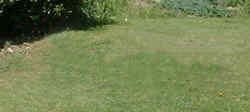# 零## 概念

两条狗## 零作为位置标志符

 502 这是 5百，没有十和两个一

## 零的值

### 例子：

"我有 3个橙子，我全都吃了，现在我有个橙子……！"

7 + 0 = 7

7 加 0 等于 7

## 特别属性

a + 0 = a 4 + 0 = 4
a − 0 = a 4 − 0 = 4
a × 0 = 0 6 × 0 = 0
0 / a = 0 0/3 = 0
a / 0 = 未定义的（除以零是未定义的 7/0 = 未定义的
0a = 0（a 为正数） 04 = 0
00 = 不确定的 00 = 不确定的
0a = 未定义的（a 为负数） 0-2 = 未定义的
0! = 1（"!" 是 阶层函数 0! = 1